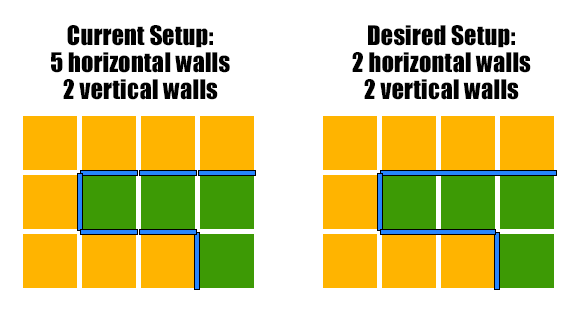# How to draw walls around rooms using the least number of objects for walls?

I have created a new project to test in before I implement it into my game. Essentially my end game will have a series of rooms. I want to generate all the walls around each room via code. I would really appreciate some help in working this problem out. The current code I have does the follow:

1. Randomly generate a floor tile from 1 to 3

2. Place an coloured object to indicate the floor tile’s number

3. Place a along wall on the north, south, east and west sides of the base cube

4. Loop through each tile and work out if the one before (either in x for horizontal, or y for vertical) and place a wall if the tile before is different than the current one.

What I want to do to optimise this all is only use a single object for continuous sides. For example: In the current code, a wall may consist of 3 single wall items in a row one after the other. I want it to just make 1 wall instead to replace these 3 walls.

So basically optimising it this way:Here is what my current setup looks like:

And here is the code I have for the above current setup:

``````using System.Collections;
using System.Collections.Generic;
using UnityEngine;

public class Walls : MonoBehaviour {

int[,] tiles;
public GameObject wall;
public Transform walls;

public GameObject floor1;
public GameObject floor2;
public GameObject floor3;
public GameObject floor;

public int width = 30;
public int height = 30;

void Start () {
tiles = new int[width,height];
GenerateTiles();
GenerateWalls();
}

void GenerateTiles() {
for(int x = 0; x < width; x++) {
for(int y = 0; y < height; y++) {
tiles[x,y] = Random.Range(1,4);
}
}
}

void GenerateWalls() {
Vector3 horizontal = new Vector3(0,0,0);
Vector3 vertical = new Vector3(0,90,0);
GameObject northWall = Instantiate(wall,new Vector3(0,-0.5f,(height / 2)),Quaternion.Euler(horizontal),walls);
northWall.transform.localScale += new Vector3(width - 1,0,0);
GameObject southWall = Instantiate(wall,new Vector3(0,-0.5f,-(height / 2)),Quaternion.Euler(horizontal),walls);
southWall.transform.localScale += new Vector3(width - 1,0,0);
GameObject eastWall = Instantiate(wall,new Vector3((width / 2),-0.5f,0),Quaternion.Euler(vertical),walls);
eastWall.transform.localScale += new Vector3(width - 1,0,0);
GameObject westWall = Instantiate(wall,new Vector3(-(width / 2),-0.5f,0),Quaternion.Euler(vertical),walls);
westWall.transform.localScale += new Vector3(width - 1,0,0);
for(int x = 0; x < width; x++) {
for(int y = 0; y < height; y++) {
if(tiles[x,y] == 1) {
GameObject newFloorTile = Instantiate(floor1,new Vector3(x - (width/2) + 0.5f,-0.5f,y - (height/2) + 0.5f),transform.rotation,floor.transform);
}
if(tiles[x,y] == 2) {
GameObject newFloorTile = Instantiate(floor2,new Vector3(x - (width / 2) + 0.5f,-0.5f,y - (height / 2) + 0.5f),transform.rotation,floor.transform);
}
if(tiles[x,y] == 3) {
GameObject newFloorTile = Instantiate(floor3,new Vector3(x - (width / 2) + 0.5f,-0.5f,y - (height / 2) + 0.5f),transform.rotation,floor.transform);
}
if(y != 0 && y != height) {
if(tiles[x,y-1] != tiles[x,y]) {
GameObject xWall = Instantiate(wall,new Vector3(x - (width / 2) + 0.5f,-0.5f,y - (height / 2)),Quaternion.Euler(horizontal),walls);
}
}
if(x != 0 && x != width) {
if(tiles[x - 1,y] != tiles[x,y]) {
GameObject yWall = Instantiate(wall,new Vector3(x - (width / 2),-0.5f,y - (height / 2) + 0.5f),Quaternion.Euler(vertical),walls);
}
}
}
}
}
}
``````

Here’s an idea for an algorithm.
While the code is recording a stretch of walls facing in the same direction, record the number of walls being placed in an integer. Once you get to a place where the walls turn, erase all of the walls placed. Then, create a new wall that is the length of each wall multiplied by the number of walls, at a position halfway down the line of walls.